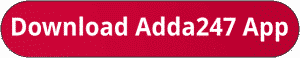Latest Banking jobs   »   sbi quant

# Quantitative Aptitude Quiz For SBI Clerk Prelims 2023-06th October

Q1. Circumference of a circle is 44 cm and radius of a cylinder is 500/7% of the diameter of the circle. If height of the cylinder is 14 cm, then find the volume (in cm³) of the cylinder?
(a) 4400 cm³
(b) 4290 cm³
(c) 4620 cm³
(d) 4200 cm³
(e) 4070 cm³

Q2. P & Q stared a business by investing Rs.5400 and Rs.4500 respectively. After four months P withdrew Rs.400 from his initial investment. If after one year the profit share of P is Rs.9240, then find the total profit?
(a) Rs.17480
(b) Rs.17340
(c) Rs.17240
(d) Rs.17640
(e) Rs.17260

Q3. The present age of Y is 28 years and four years hence the ratio of age of X to Y will be 7: 8. If present age of Z is 25% more than the present age of X, then find the age of Z six years ago?
(a) 26 years
(b) 22 years
(c) 20 years
(d) 24 years
(e) 28 years

Q4. Pipe P & Q together can fill a tank in 20 minutes, while pipe P, Q and R together can fill the same tank in 25 minutes. If Pipe Q & R together can fill the tank in 50 minutes, then in how many minutes pipe P alone can fill the same tank completely?
(a) 75 min
(b) 30 min
(c) 40 min
(d) 60 min
(e) 50 min

Q5. A train crosses a man running in opposite direction in 12 seconds. If speed of man and speed of train is 24 km/hr & 84 km/hr respectively, then find the length of train (in meters)?
(a) 324
(b) 360
(c) 344
(d) 380
(e) 310

Q6. The difference between marked price and selling price of an article is Rs.84 and the marked price of the article is 40% more than its cost price. If shopkeeper allowed 30% discount, then find the cost price of the article.
(a) Rs.250
(b) Rs.400
(c) Rs.150
(d) Rs.200
(e) Rs.450

Q7. 180 liters of mixture of milk and water is in the ratio of 7:5 respectively. If 15-liter milk and Y liters of water added in the mixture, then the ratio of milk to water becomes 6:5 respectively. Find the value of Y.
(a) 20
(b) 5
(c) 15
(d) 10
(e) 25

Q8. P is 25% more efficient than Q and R is 40% less efficient than P. If P and R together can complete a work in 12 days, then find the time taken by P, Q & R together to complete the same work.
(a) 12 days
(b) 6 days
(c) 9 days
(d) 8 days
(e) 15 days

Q9. X, Y and Z started a business by investing of Rs.2400, Rs.3000 and Rs.2600 respectively. After four months, X and Y withdrew his investment by 25% and 20% respectively. If at the end of the years Z received profit of Rs.1950, then find the profit share of X.
(a) Rs.1250
(b) Rs.1500
(c) Rs.1850
(d) Rs.1900
(e) Rs.1000

Q10. Ratio of present ages of A to B is 5: 4 and present age of C is 40% more than that of A. If the average of present ages of A, B & C is 16 years, then find the age of B six years ago.
(a) 6 years
(b) 15 years
(c) 8 years
(d) 12 years
(e) 4 years

Solutions. .               .                                      .       .

## FAQs

### What is the selection process of SBI Clerk 2023?

The selection process of SBI Clerk 2023 consists of Prelims, Mains and LPT.

#### Congratulations!Union Budget 2023-24: Free PDF